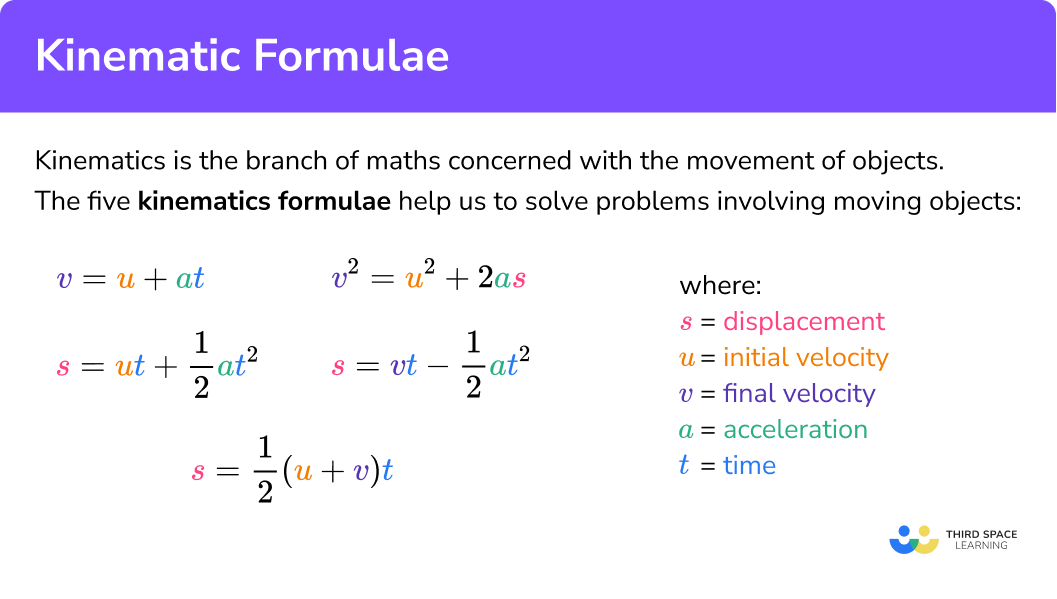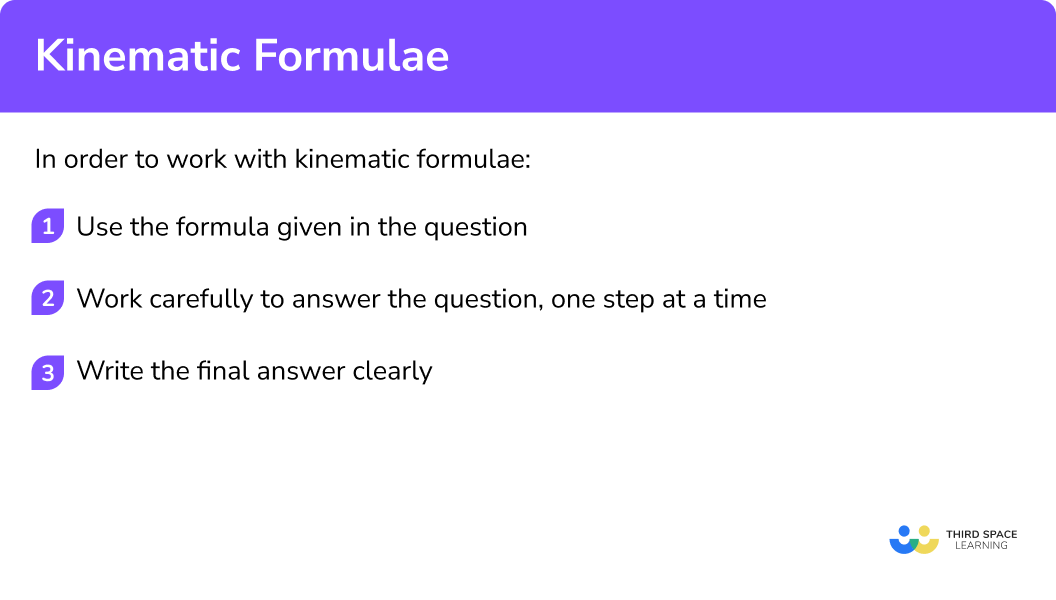GCSE Maths Algebra Maths Formulas

Kinematics Formula

# Kinematic Formulae

Here we will learn about the kinematics formula, including what they are and how to use them to solve kinematics problems.

There are also kinematics formula worksheets based on Edexcel, AQA and OCR exam questions, along with further guidance on where to go next if you’re still stuck.

## What is a kinematics formula?

The kinematics formula are used to solve problems involving moving objects. Kinematics is the maths concerned with the movement of objects.

To do this we need to introduce the 5 different kinematic formulas. They involve 5 different variables of motion. Velocity is speed in a given direction. Displacement is the distance from the original position. In all kinematics formulas,

s= displacement

u= initial velocity

v= final velocity

a= acceleration

t= time

The kinematics formulas are,

\begin{aligned} & v=u+at \\\\ &s=ut+\cfrac{1}{2}at^2 \\\\ &s=vt-\cfrac{1}{2}at^2 \\\\ &s=\cfrac{1}{2}(u+v)t \\\\ &v^2=u^2+2as \end{aligned}

The kinematic formulas are sometimes known as the suvat equations. They are also known as equations of motion.

These kinematic formulas are used when there is constant acceleration. Kinematics when the acceleration is not constant is covered at A Level. We do not need to worry about other factors affecting motion of an object such as air resistance.

The kinematic formulas are based on Newton’s laws of motion. You may also come across these formulas in physics topics in science.

The units which are usually used in kinematics are m (metres) for displacement, s (seconds) for time, m/s (metres per second) for velocity and m/s^2 (metres per second squared) for acceleration.

When an object is slowing down it has a negative acceleration. This is known as deceleration.

### What is a kinematics formula?## How to work with kinematic formulae

In order to work with kinematic formulas:

1. Use the formula given in the question.
2. Work carefully to answer the question, one step at a time.
3. Write the final answer clearly.

### Explain how to work with kinematic formulae### Related lessons on maths formulas

Kinematic formulae is part of our series of lessons to support revision on maths formulas. You may find it helpful to start with the main maths formulas lesson for a summary of what to expect, or use the step by step guides below for further detail on individual topics. Other lessons in this series include:

## Kinematics formula examples

### Example 1: substituting values

Given that s=\cfrac{1}{2}(u+v)t, work out s, when u=5, \ v=20 and t=4.

1. Use the formula given in the question.

The formula is

s=\cfrac{1}{2}(u+v)t.

2Work carefully to answer the question, one step at a time.

Substitute the values into the formula.

\begin{aligned} s&=\cfrac{1}{2}(u+v)t \\\\ s&=\cfrac{1}{2}\times (5+20)\times 4 \\\\ s&= 50 \end{aligned}

s= 50.

### Example 2: rearranging a kinematic formula

Rearrange v=u+at to make a the subject.

The formula is

v=u+at.

We need to rearrange the formula, one step at a time using inverse operations.

\begin{aligned} v&=u+at \\\\ v-u&=at \\\\ \cfrac{v-u}{t}&= a \end{aligned}

a=\cfrac{v-u}{t}.

### Example 3: solving kinematic equations

For the following question you may use the formula v^2=u^2+2as.

Calculate s when v is 10, \ u is 0 and a is 20.

The formula given in the question is

v^2=u^2+2as.

First we substitute the values into the kinematic formula,.

\begin{aligned} v^2&=u^2+2as\\\\ 10^2&=0^2+2\times 20\times s\\\\ 100&=0+40s \end{aligned}

Then we can rearrange to solve for s.

\begin{aligned} 100&=0+40s\\\\ 100&=40s\\\\ \cfrac{100}{40}&=s\\\\ 2.5&=s \end{aligned}

s=2.5.

### Example 4: solving a kinematic problem

For the following question you may use the formula s=ut+ \cfrac{1}{2}at^2 where

t= time u= initial velocity

a= acceleration s= displacement

A car is stopped at a traffic light. When the light goes green the car immediately accelerates at 5 \ m/s^{2}. How far does the car travel in 10 seconds?

The formula given is

s=ut+\cfrac{1}{2}at^2.

The initial velocity u is 0 as the car is stationary at the start of the kinematics situation. We substitute the values into the kinematic formula.

\begin{aligned} s&=ut+\cfrac{1}{2}at^2 \\\\ s&=0 \times 10+ \cfrac{1}{2} \times 5 \times 10^2 \\\\ s&=250 \end{aligned}

The final answer is, the car has travelled 250m in 10 seconds.

### Common misconceptions

• Acceleration can be negative

You may be asked to work out the acceleration of a situation involving the motion of an object. Acceleration can be negative. When this happens the object is slowing down. This is known as deceleration.

• Take care with \textbf{v} and \textbf{u}

The variables v and u can easily be muddled as they look similar and they both represent velocity. u is the initial velocity; the velocity at the beginning of the situation. v is the final velocity; the velocity at the end of the situation.

• Units

The units which are mostly used in kinematics are m (metres) for displacement, s (seconds) for time, m/s (metres per second) for velocity and m/s^2 (metres per second squared) for acceleration. Make sure the units in your answer are consistent with those of the given values

• Initial velocity

Sometimes you are told the objects starts at rest, this means that the initial velocity is 0 \ m/s.

### Practice kinematic formulae questions

1. Given that v=u+at, find v when u=50, \ a=-10, \ t=4.

10903070Substitute the values into the equation and work out the calculation.

\begin{aligned} v&=u+at \\\\ v&=50+(-10)\times 4 \\\\ v&=50-40\\\\ v&= 10 \end{aligned}

2. Given that s=\cfrac{1}{2}(u+v)t, calculate s when u=10, \ v=80, and t=6.

5401530270Substitute the values into the equation and work out the calculation.

\begin{aligned} s&=\cfrac{1}{2}(u+v)t \\\\ s&=\cfrac{1}{2}\times (10+80)\times 6\\\\ s&=\cfrac{1}{2}\times 90\times 6\\\\ s&= 270 \end{aligned}

3. Rearrange v^2=u^2+2as to make s the subject.

s=\cfrac{v^2+2a}{u^2}s=\cfrac{v^2-u^2}{2a}s=\cfrac{v^2+u^2}{2a}s=\cfrac{v^2-2a}{u^2}Rearrange, taking one-step at a time.

\begin{aligned} v^2&=u^2 +2as\\\\ v^2-u^2&=2as \\\\ \cfrac{v^2-u^2}{2a}&=s \end{aligned}

4. Given that v=u+at, find u when v=200, \ a=20, \ t=3.

180300140260Substitute the given values into the formula, then solve to find the unknown variable u.

\begin{aligned} v&=u+at \\\\ 200&=u+20\times 3 \\\\ 200&= u+60\\\\ u&=140 \end{aligned}

5. A ball is dropped from a tower and is in free fall. Its initial velocity is 0 \ m/s. The gravitational acceleration is 10 \ m/s^{2}. The final velocity as the ball hits the ground is 50 \ m/s. Calculate the time the ball was in free fall. You may use the formula v=u+at.

40 seconds5 seconds40 minutes10 minutesSubstitute the given values into the formula, then rearrange to solve to find the unknown variable t.

\begin{aligned} v&=u+at \\\\ 50&=0+10\times t \\\\ 50&= 10t\\\\ t&=5 \end{aligned}

The answer will be 5 seconds, as seconds is consistent with the other units.

6. A train is travelling at 30 \ m/s. It brakes for 3 seconds and it decelerates at 5 \ m/s^{2}. Calculate how far the train travels in those 3 seconds. You may use the formula s=ut+\cfrac{1}{2}at^2.

67.5 \ m112.5 \ m82.5 \ m97.5 \ mSubstitute the given values into the formula.

\begin{aligned} s&=ut+\cfrac{1}{2}at^2 \\\\ s&=30\times 3+\frac{1}{2}\times (-5) \times 3^2\\\\ s&=90-22.5 \\\\ s&=67.5 \end{aligned}

The answer will be 67.5 metres, as metres is consistent with the other units.

### Kinematics formula GCSE questions

1. Given s=vt-\cfrac{1}{2}at^2 calculate s when v=50, \ a=10 and t=2.

(2 marks)

s=50\times 2-\cfrac{1}{2}\times 10\times 2^2

(1)

s=80

(1)

2. Rearrange v^2=u^2+2as to make a the subject.

(2 marks)

v^2-u^2=2as

(1)

a=\cfrac{v^2-u^2}{2s}

(1)

3. For the following question you may use the formula v=u+at where

v= final velocity u= initial velocity

a= acceleration t= time

A motorbike is travelling along a road at a velocity of 15 \ m/s. The motorbike accelerates at 2 \ m/s^2 for 5 seconds. Find the final velocity of the motorbike.

(2 marks)

v=15+2\times 5

(1)

v=25 \ m/s

(1)

## Learning checklist

You have now learned how to:

• Use kinematics formula
• Problem solve using kinematics formula
• Rearrange kinematic equations

## Still stuck?

Prepare your KS4 students for maths GCSEs success with Third Space Learning. Weekly online one to one GCSE maths revision lessons delivered by expert maths tutors.

Find out more about our GCSE maths tuition programme.